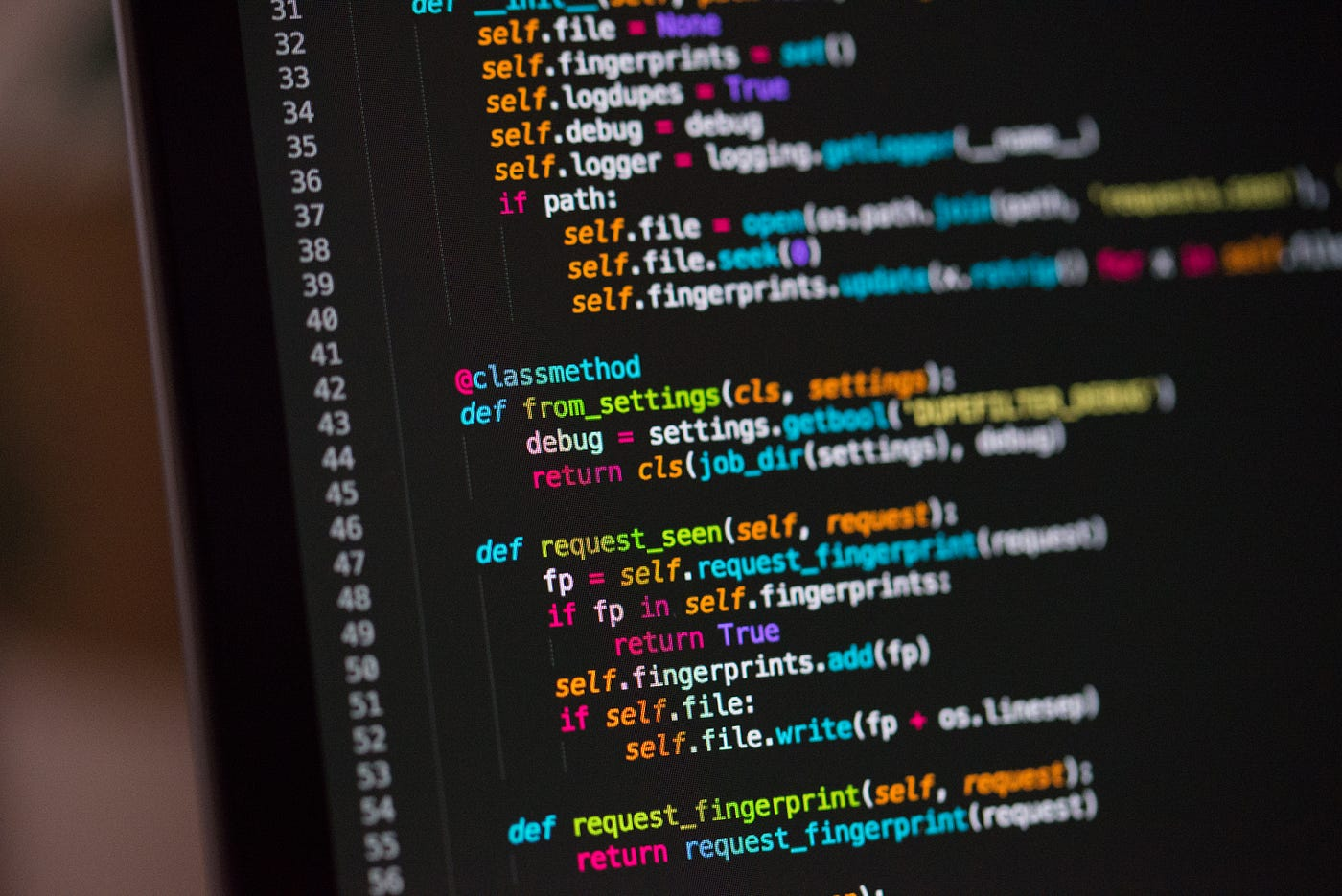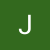# The Blind 75 Leetcode Series: Best Time to Buy and Sell StockPhoto by Chris Ried on Unsplash

Today, we are working on 121. Best Time to Buy and Sell Stock

You are given an array `prices` where `prices[i]` is the price of a given stock on the `ith` day.

You want to maximize your profit by choosing a single day to buy one stock and choosing a different day in the future to sell that stock.

Return the maximum profit you can achieve from this transaction. If you cannot achieve any profit, return `0`.

We have a list of numbers indicating stock price. We want to achieve the max profit by buying on one day and selling on another. The buying time, of course, has to be before the selling time.

Some questions to further specify the problem would be

1. are we dealing with only integers?
2. Is there a limit on number range?
3. following the above, do we expect negative numbers?

If the interviewers tell us that we only expect non-negative integers in the list and there are always going to be some elements in the list, then we ca start.

The brute force approach is of course checking every single combination of numbers and keep updating the max. This can be done with some for-loops like

`def maxProfit(prices):    max_profit = 0    for i in range(len(prices)-1):        for j in range(i+1, len(prices)):            max_profit = max(max_profit, prices[j] - prices[i])    return max_profit`

This works, but time complexity would be O(N²) which is not preferred. If you’ve been following this series, you should be able to tell that a double for-loop like this might be replaced by 2 pointers, and we can reduce the time complexity for a magnitude of N.

But how do we move the 2 pointers? What conditions do we set for the script to know when to move the left pointer and when to move the right one?

Let’s look at the problem again. We want to find max profit, so naturally we want to find a max stock price and a min stock price. Taking a diff of these two can get us the max profit. However, we want to make sure that the max values only come from the the days after our min value, so the value becomes localized.

We want to keep our left pointer at minimum to maximize the profit, so every time we see a value on the right pointer that’s less than the left, we move the left to the right. Otherwise, we compare the diff with the previous max profit and find a new one.

So the code looks like this

`def maxProfit(prices):    max_profit = 0    n = len(prices)    if n <=1:        return max_profit    i, j = 0, 1    while j <= n - 1:        if prices[j] > prices[i]:            max_profit = max(max_profit, prices[j] - prices[i])        else:            i = j        j += 1    return max_profit`

What’s the time complexity now? We go through the list once only, so we are looking at O(N). Quite an improvement.

`def maxProfit(prices):    max_profit = 0    n = len(prices)    if n <=1:        return max_profit    i, j = 0, 1    while j <= n - 1:  # O(N)        if prices[j] > prices[i]:            max_profit = max(max_profit, prices[j] - prices[i])  # O(1)        else:            i = j        j += 1    return max_profit`

This one is a lot easier in my opinion compare to the previous ones. The difficulty for this is indeed easy but I can see people getting stuck on figuring out when to move the pointers. We just need to make sure we are buying on the local minimum and want to find a max point from later time by calculating the max diff at each step.

That’s it! Another problem down.